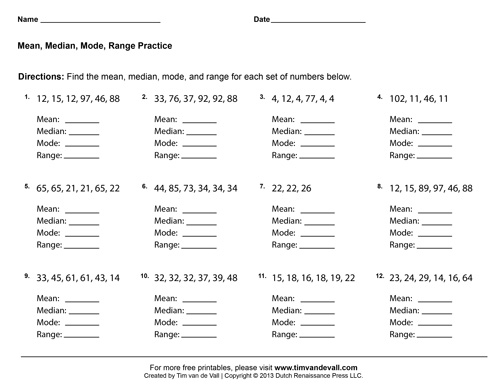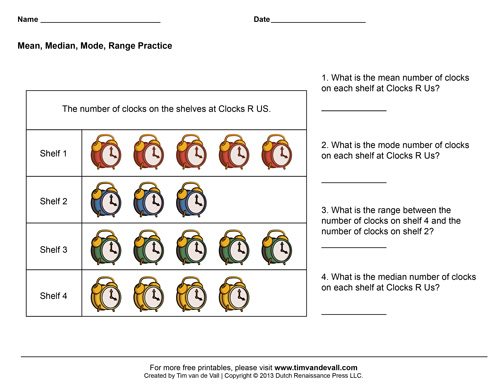# Mean Median Mode Range Worksheets – Sixth Grade Math Worksheets

On this page you’ll find a set of three printable Mean, Median, Mode and Range worksheets for Sixth Grade Math students and teachers. In the first two worksheets, students have to find the mean, median, mode, and range for each set of numbers. For the third worksheet, students have to answer questions based on the table.

## Mean Median Mode Range Worksheets